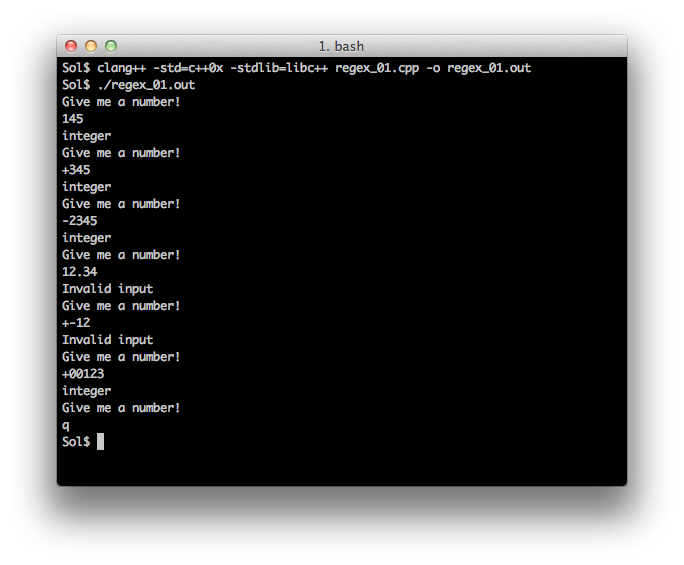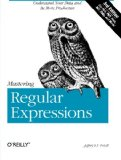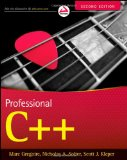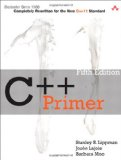# C++11 regex tutorial

## Posted on October 12, 2011 by Paul

The code for this tutorial is on GitHub: https://github.com/sol-prog/regex_tutorial.

Now that C++11 is a published standard and most of the mainstream compilers already have some of the C++11 standard implemented it is time to learn or relearn what has practically became a new language. As I explore myself some of the newest features of the standard, I plan to blog about my progress and hopefully help other people to start learning C++11.

I will start a series of short tutorials in which I will present one feature of the language at a time illustrated with working, complete, examples. Each post will also have a short paragraph about on which compilers and operating system I've tested my code. I will test each example on Linux (g++-4.6.1 and clang), Mac OSX (g++-4.6.1 and clang) and finally Windows (Visual C++ 2010 and g++-4.6.1). If you find that one of the examples presented here works with a different combination of compiler/OS, please drop me a note and I will include this in my post.

Validating the user input was always a problem in C++, especially when the user is supposed to enter a number. Each programmer was forced to write his own error checking functions for numerical input validation, basically it was no uniform syntax for checking the correctness of the user input. Enter C++11 and regular expression! Now it is possible to check in a simple and effective way if the input is what you as a coder expect from the user.

C++11 has suport for a few regular expression grammars like ECMAScript, awk, grep and a few others, all examples from this tutorial use the ECMAScript syntax. In order to use the regex capabilities of C++11 you will need to know (or to be wiling to learn) the ECMAScript grammar.

Let's start with a simple example, suppose we are trying to validate an integer inputed by the user. C++ will accept this kind of numerical input: 0012, 12, +0012 or the negative -0012, -12, -0012. Any leading zeros will be ignored and for positive numbers we can safely use, or not, the plus sign.

First we will need a regular expression that will match numbers in the above format. According to ECMAScript a digit is identified with [:digit:] or with [:d:]. A regular expression that will match any one digit number is (using C++11 syntax):

The above will match any input of the form 0 .. 9. If you want to match a number larger than one digit (a string of digits) you will use the plus sign at the end of the above regex:

What about a signed number like -12 or +12 ? With the minus sign we can simply test if the first position in the input is minus or not. This can be achieved by using -? in front of our regular expression, now we will have:

The plus sign is a special character in the ECMAScript syntax, so you need to inform the compiler to treat plus as the character + and not as a repetition operator, this can be done by "escaping" the special character with \ , however \ is itself a special character so we will end up writing:

Now, all we have to do is to combine the last two expressions, basically a number can have only a minus or a plus sign, so we need to use the "or" operator which for ECMAScript is the character | . We will group the "+ or -" expression using a pair of parentheses:

Let's wrap the above expression in a simple text based program that will ask for an integer until the user inputs "q". If we have a match between our regex and the input we will print "integer":

Save the above code in a file named "regex_01.cpp". In order to compile the above code on a Mac OSX machine with Xcode we will use:

Running the above code:At the time of this writing gcc has no support for regex, the only compilers that can compile the above code (after my knowledge) are clang and Visual C++ 2010.

What about testing for a real number ? First we will construct a regex that will match only these kind of formats: -x, -x., -x.xx and so on. We can use as a starting point the integer regular expression, for the fractional part of a real number we will obviously use a similar expression without the sign part: [[:digit:]]+ .

A real number can be inputed without a fractional part, we need to mark the fractional part as "optional" in our expression, this can be written as ([[:digit:]]+)? . Also, the decimal separator must be optional, a regular expression for a real number can be written as:

You can find a complete example with the above regex in "regex_02.cpp" on github.

Let's construct a regular expression that can be used to match numbers written in scientific format e.g. -1.23e+06, 0.245e10, 1E5. We will start with these observations: the exponential part is optional, the sign is also optional. The first part of our regular expression will obviously be the one used earlier, for the exponential part we will use ((e|E)((\\+|-)?)[[:digit:]]+)? :

An example with the above regex is on github, the file is named "regex_03.cpp".

Similar expressions can be constructed for testing any kind of user input. If you want to learn more about regular expressions, the most authoritative source in the filed is the book Mastering Regular Expressions by Jeffrey E.F. Friedl:If you are interested in learning more about the new C++11 syntax I would recommend reading Professional C++ by M. Gregoire, N. A. Solter, S. J. Kleper 2nd edition:or, if you are a C++ beginner you could read C++ Primer (5th Edition) by S. B. Lippman, J. Lajoie, B. E. Moo.In the next regex tutorial I will show you how to use regular expressions to clean a text, e.g. you can clean a text from all html tags or you can search for spelling errors.